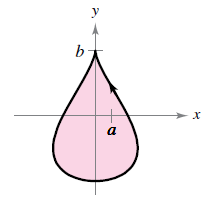Chapter 10.3, Problem 86E

Chapter
Section
Textbook Problem

# Areas of Simple Closed Curves In Exercises 81-86, use a computer algebra system and the result of Exercise 77 to match the closed curse with its area. (These exercises were based on “The Surveyor’s Area Formula'’ by Bart Braden, College Mathematics Journal, September 1986, pp. 335-337, by permission of the author.)(a) 8 3 a b (b) 3 8 π a 2 (c) 2 π a 2 (d) π a b (e) 2 π a b (f) 6 π a 2 Teardrop: ( 0 ≤ t ≤ 2 π ) x = 2 a cos t − a sin 2 t y = b sin tTo determine

To calculate: The close curve area of a teardrop, x=2acostasin2t and y=bsint in the interval 0t2π with graphing utility and match the result with the given areas.

Explanation

Given:

Parametric equations are, x=2acostasin2t,y=bsint and the figure:

Formula used:

If y is a continuous function of x on the interval axb, where x=f(t) and y=g(t), then,

|abydx|=|t1t2g(t)f(t)dt| where f(t1)=a and f(t2)=b and both g and f are continuous on [t1,t2].

Calculation:

Consider the equation of the teardrop, x=2acostasin2t,y=bsint.

Assume that f(t)=x and g(t)=y.

Now use the formula, |abydx|=|t1t2g(t)f(t)dt| to find the area of the teardrop,

So, differentiate f(t) with respect to t

### Still sussing out bartleby?

Check out a sample textbook solution.

See a sample solution

#### The Solution to Your Study Problems

Bartleby provides explanations to thousands of textbook problems written by our experts, many with advanced degrees!

Get Started

#### Explain in your own words the meaning of limxf(x)=L and limxf(x)=M.

Applied Calculus for the Managerial, Life, and Social Sciences: A Brief Approach

#### In problems 37-48, compute and simplify so that only positive exponents remain. 41.

Mathematical Applications for the Management, Life, and Social Sciences

#### (i · j)k = k 2-k 0 (the number 0) 0 (the zero vector)

Study Guide for Stewart's Multivariable Calculus, 8th

#### For , f′(x) =

Study Guide for Stewart's Single Variable Calculus: Early Transcendentals, 8th